SYLLABUS  Previous: 3.4 Hedging an option  Up: 3 FORECASTING WITH UNCERTAINTY  Next: 3.6 Computer quiz

3.5 Hedging a bond with another bond (Vasicek)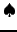[ SLIDE Ito - no random - no arbitrage - Vasicek - price of risk || VIDEO modem - LAN - DSL]

In contrast to the price of a share (which can never drop below zero because of the regulations of bankruptcies), it is in principle possible to live with negative interest rates: these have even been observed in Switzerland in the 1960s, albeit only for a short period. This motivates the development of stochastic models for long term interest rates where the random walk of the spot rate increments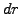is based on (3.3.1#eq.1) and the distribution chosen somewhere between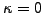(normal walk assumed by Vasicek ) and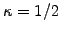(assumed by Cox, Ingersoll and Ross ).
Here we follow the classical derivation from Vasicek usingand apply Itô's lemma to calculate the stochastic price increment for a bond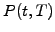of maturity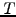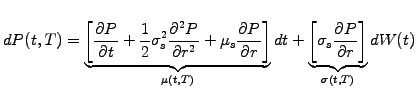(3.5#eq.1)

The drift and volatility of a bond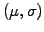depend on the spot rate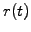and can be parametrized using market data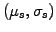. Having no anti-correlated underlying as for the case of stock options, the trick here is to create here a portfolio that is long one bond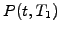and short a number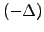of bonds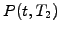with a different maturity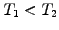. The portfolio value and its incremental change per time step become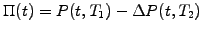(3.5#eq.2)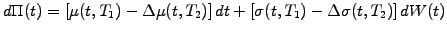(3.5#eq.3)

Choosing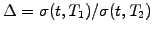to eliminate the random component, the portfolio becomes deterministic and, using no-arbitrage arguments, earns exactly the risk-free spot rate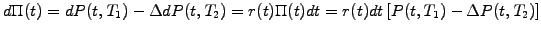(3.5#eq.4)

Substitute the value for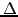, insert (3.5#eq.1) for the increments and move all the terms with the same maturity on the same side of the equation to obtain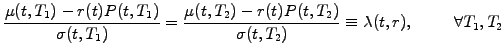(3.5#eq.5)

This shows that the so-called market price of risk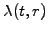is independent of the maturity and can therefore be used to parameterize the market. Rewriting the bond drift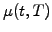in (3.5#eq.1) in terms of the market price of risk (3.5#eq.5), the properly hedged portfolio (3.5#eq.2) finally yields the bond pricing equation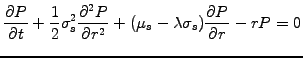(3.5#eq.6)

which can be solved using the normalized terminal payoff at maturity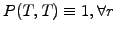as the terminal condition. Boundary conditions depend on the model for the spot rate, e.g.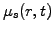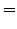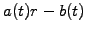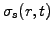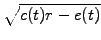(3.5#eq.7)

which can be used with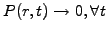when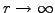and keeping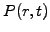finite for small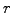.
Using (3.5#eq.6) to re-write the deterministic component of a single bond (3.5#eq.1), Itô's lemma can be put into another form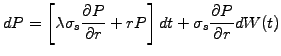(3.5#eq.8)

or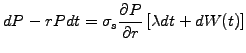(3.5#eq.9)

showing on the left hand side that a higher return can be earned exceeding the risk-free interest rates, provided that the investor accepts a certain level of risk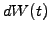. Indeed, the portfolio grows by an extra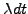per unit of risk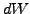. This justifies the interpretation of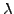as the market price of risk, with investors that are either risk seeking or risk averse depending whetheris positive or negative.

SYLLABUS  Previous: 3.4 Hedging an option  Up: 3 FORECASTING WITH UNCERTAINTY  Next: 3.6 Computer quiz